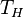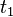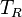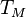# Risk Horizon

## Definition

Risk Horizon ($T_H$) denotes a future time point (period) that forms the focus of a Risk Analysis exercise or calculation. In the context of a Credit Network model, a risk horizon is any future timepoint at which the overall external and internal state and balance sheet is assessed by conditioning on the modeled forward states at that timepoint (and all prior points when non-Markovian behavior is modeled).

## Usage

The analysis of residual liability risk expectations at the risk horizon enables the framework to support Going Concern Risk Capital analyses.

The choice of risk horizon is rather arbitrary. It tends to mirror the time-scale of processes and risk factors. Guidance for selecting the risk horizon is provided by the timescale over which the portfolio exhibits significant change. There are natural choices with respective pros and cons:

• One year horizon aligned with i) the 12m period required for SICR evaluation and ii) the 1yr risk horizon embedded in advanced internal rating based models for Basel II/III Regulatory Capital. This choice requires that loss allowance and ECL is estimated for each scenario at the end of the year. Aligned with the annual Financial Accounts reporting cycle (helps managing the uncertainty around the next

reporting period)

• Three to Five years risk horizon, aligned with the risk horizon of regulatory stress testing. Residual ECL at this longer risk horizon still needs to be estimated. The calculations at intermediate periods (e.g 1 yr, 2 yr) must grapple with the fact that at those points there is still uncertainty. One technique is the perfect foresight assumption. It amounts to equating forward expectations conditional to a scenario up to point$t_1$ to the outcomes of the single scenario continuation up to$T_R$. This choice ignores any impact of management actions over a long period (potentially unrealistic) and must make assumptions about portfolio replenishment for significant fractions of the portfolio (all assets with less than risk horizon maturity).
• Another option is "hold-to-maturity" of the portfolio. This is not conceptually fit for very long maturity portfolios as it further exacerbates the previous points

## Issues and Challenges

• When the risk horizon is less than the final horizon ($T_M$) there are residual risks that must be assessed at$T_H$. This can be done by a number of distinct approaches
• Perfect Foresight Models: A single scenario for residual risk realization which may be the most likely scenario or a stressed scenario
• Expectation Models: Multiple probability weighted scenarios for residual risk realization
• The focus on a single risk horizon may be forcing simplified capture of phenomena occurring at different timescales
• While multi-period risk frameworks have been proposed, they are more difficult to incorporate into risk management